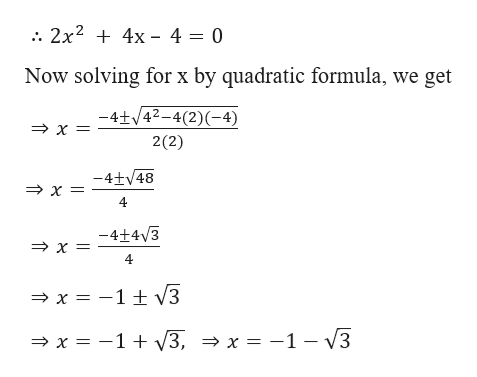# The question was: Find the horizontal intercepts of the quadratic f(x)=2x2+4x-4. I utilized the quadratic formula to arrive at my answer. I got (-1+sqrt(3),0) and (-1-sqrt(3),0). I would just like to see if this is correct.

Question

The question was: Find the horizontal intercepts of the quadratic f(x)=2x2+4x-4. I utilized the quadratic formula to arrive at my answer.

I got (-1+sqrt(3),0) and (-1-sqrt(3),0).

I would just like to see if this is correct.

check_circleExpert Solution
Step 1

Given,

f(x)= 2x2 + 4x – 4

Step 2

For horizontal interce...help_outlineImage Transcriptionclose2x2 4x 4 = 0 Now solving for x by quadratic formula, we get -4+/42-4(2)(-4) x 2(2) -4+48 x = 4 -4+4 3 x 4 > x= -1 t 3 V3, > x = -1 - V3 x 1 fullscreen

### Want to see the full answer?

See Solution

#### Want to see this answer and more?

Solutions are written by subject experts who are available 24/7. Questions are typically answered within 1 hour*

See Solution
*Response times may vary by subject and question
Tagged in

### Equations and In-equations